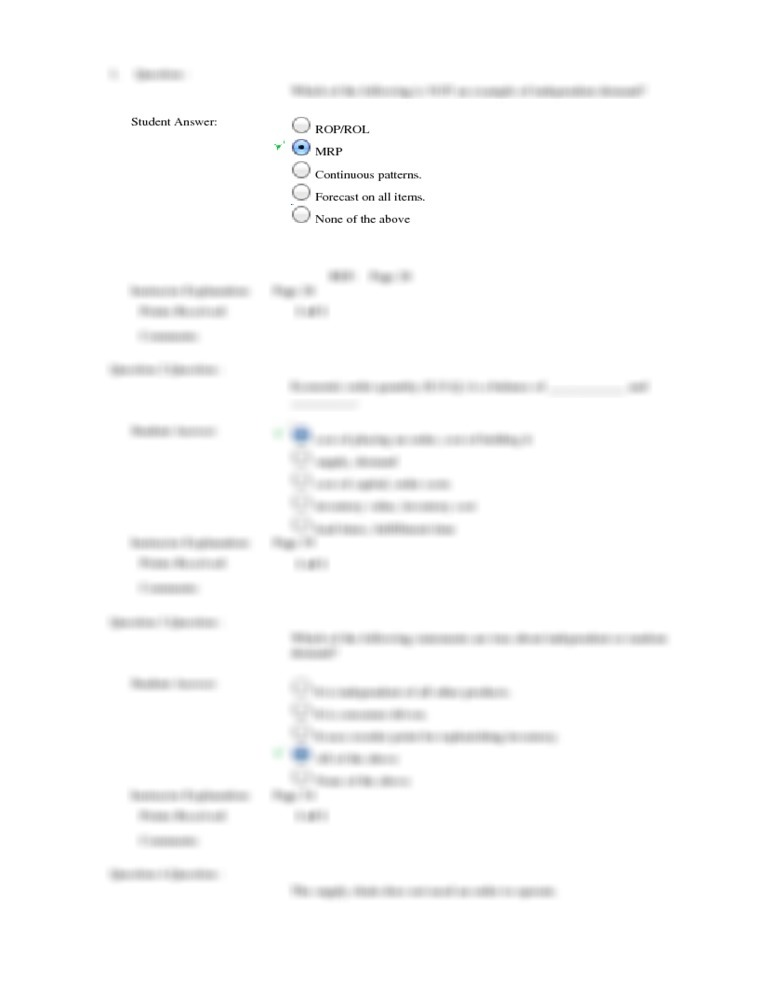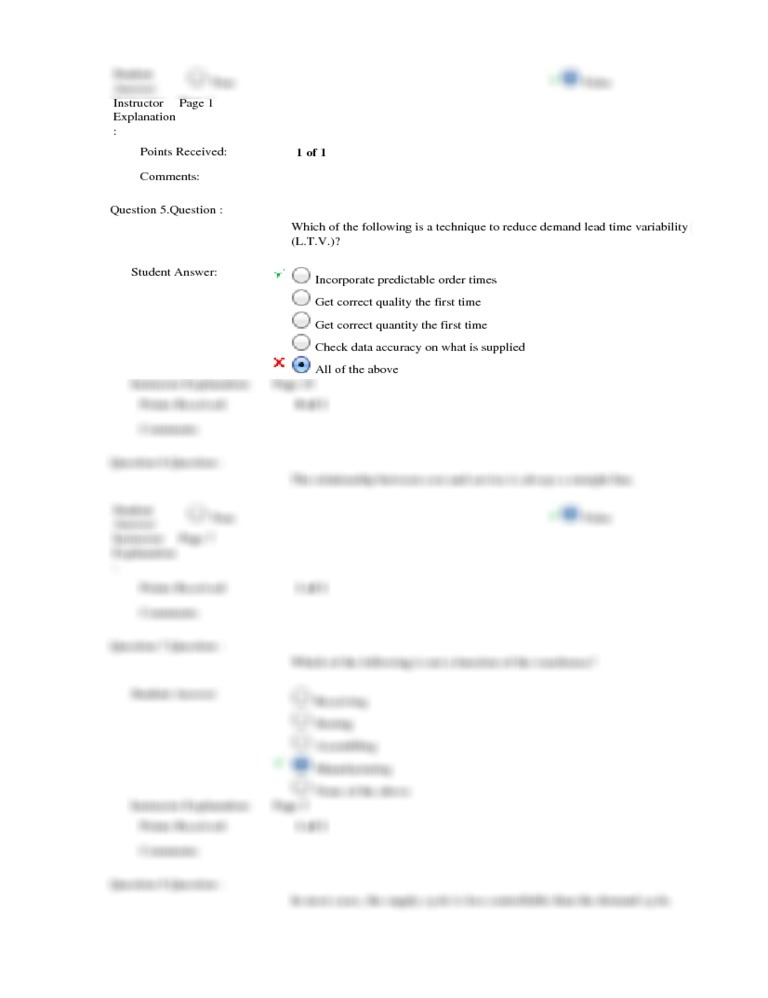Back to Search
Ashford
Strategic warehouse management
week 1
MGT 496 Week 1 Quiz

# MGT 496 Week 1 Quiz

Short description

1. Question : Which of the following is NOT an example of independent demand? Question 2. Question : Economic order quantity (E.O.Q.) is a balance of ____________ and __________. Question 3. Question : Which of the following statements are true about independent or random demand? Question 4. Question : The supply chain does not need an order to operate. Question 5. Question : Which of the following is a technique to reduce demand lead time variability (L.T.V.)? Question 6. Question : The relationship between cost and service is always a straight line. Question 7. Question : Which of the following is not a function of the warehouse? Question 8. Question : In most cases, the supply cycle is less controllable than the demand cycle. Question 9. Question : Outbound logistics covers which of the following? Question 10. Question : A supply chain can be compared to which of the following:

Document previewBack to Search